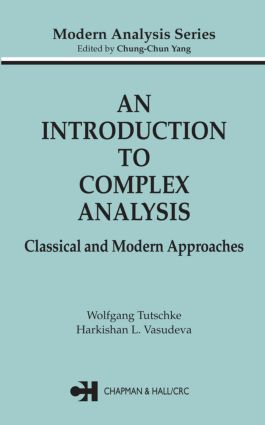# An Introduction to Complex Analysis

## Classical and Modern Approaches, 1st Edition

Chapman and Hall/CRC

480 pages | 52 B/W Illus.

##### Purchasing Options:\$ = USD
Hardback: 9781584884781
pub: 2004-06-25
Currently out of stock
\$140.00
x
eBook (VitalSource) : 9780429115066
pub: 2004-06-25
from \$28.98

FREE Standard Shipping!

### Description

Like real analysis, complex analysis has generated methods indispensable to mathematics and its applications. Exploring the interactions between these two branches, this book uses the results of real analysis to lay the foundations of complex analysis and presents a unified structure of mathematical analysis as a whole.

To set the groundwork and mitigate the difficulties newcomers often experience, An Introduction to Complex Analysis begins with a complete review of concepts and methods from real analysis, such as metric spaces and the Green-Gauss Integral Formula. The approach leads to brief, clear proofs of basic statements - a distinct advantage for those mainly interested in applications. Alternate approaches, such as Fichera's proof of the Goursat Theorem and Estermann's proof of the Cauchy's Integral Theorem, are also presented for comparison.

Discussions include holomorphic functions, the Weierstrass Convergence Theorem, analytic continuation, isolated singularities, homotopy, Residue theory, conformal mappings, special functions and boundary value problems. More than 200 examples and 150 exercises illustrate the subject matter and make this book an ideal text for university courses on complex analysis, while the comprehensive compilation of theories and succinct proofs make this an excellent volume for reference.

### Reviews

"Many things, which are briefly described in others books, in remarks or exercises, are given in full detail … . [It] will please readers interested … in applications as well as those who want to know how things really work and prefer deeper and more detailed treatment of the material. The book also contains more than 200 examples and 150 exercises. … I recommend it for courses in complex function theory … and also as a reference book."

- EMS Newsletter, Dec. 2004

"… [A]bundant examples and 'hints' to aid readers [are provided]. Summing Up: Recommended. Upper-division undergraduates through professionals."

- CHOICE, March 2005, Vol. 42, No. 07

"For the unification of the structure of mathematical analysis as a whole, it is imperative to use results of real analysis when laying the foundations of complex analysis. This is done in the present book."

-Zentralblatt MATH

PRELIMINARIES

The field of complex numbers

The complex plane

Metric spaces

Mappings and functions. Continuity

THE CLASSICAL APPROACH

Ordinary complex differentiation

Preliminaries of the Integral Calculus

Complex Integral Theorems

AN ALTERNATIVE APPROACH

Partial complex differentiations

Complex Green-Gauss Integral Theorems

Generalized Cauchy Integral Formula

The classical Cauchy Integral Formula

Comparison

LOCAL PROPERTIES

Existence of higher order derivatives

Local power series representation

Distribution of zeros

The Weierstrass Convergence Theorem

Connection with plane Potential Theory

Complex Integral Theorems revisited

GLOBAL PROPERTIES

Analytic continuation

Maximum Modulus Principle

Entire functions

Fundamental Theorem of Algebra

ISOLATED SINGULARITIES

Classification

Laurent series

Characterization by the principal part

Meromorphic functions

Behavior at essential singularities

Behavior at infinity

Partial fractions of rational functions

Meromorphic functions on the Sphere

HOMOTOPY

Statement of the problem

Homotopic curves

Path independent line integrals

Simply connected domains

Solution of first order systems

Conjugate solutions

Inversion of complex differentiation

Morera's Theorem

Potentials of vector fields

RESIDUE THEORY

Statement of the problem

Winding numbers

The integration of principal parts

Residue Theorem

Calculation of residues

APPLICATIONS OF RESIDUE CALCULUS

Total number of zeros and poles

Evaluation of definite integrals

Sum of certain series

MAPPING PROPERTIES

Continuously differentiable mappings

Conformal mappings

Examples of conformal mappings

Univalent functions

Riemann's Mapping Theorem

Construction of flow lines

SPECIAL FUNCTIONS

Prescribed principal parts

Prescribed zeros

Infinite products

Weierstrass products

Gamma Function

The Riemann Zeta Function

Elliptic Functions

BOUNDARY VALUE PROBLEMS

Preliminaries

The Poisson Integral Formula

Cauchy Type Integrals

Desired Holomorphic Functions

### Subject Categories

##### BISAC Subject Codes/Headings:
MAT003000
MATHEMATICS / Applied
MAT037000
MATHEMATICS / Functional Analysis